Here we are providing Class 11 chemistry Important Extra Questions and Answers Chapter 2 Structure of Atom Chemistry. Chemistry Class 11 Important Questions are the best resource for students which helps in Class 11 board exams.

## Class 11 Chemistry Chapter 2 Important Extra Questions Structure of Atom Chemistry

### Structure of Atom Chemistry Important Extra Questions Very Short Answer Type

Question 1.
How many total electrons are present in nitrate ion?
No. of electrons in NO3 ion
= No. of electrons on N + No. of electrons on 3 oxygen atom + one ē
= 7 + 3 × 8 + 1 = 32 electrons.

Question 2.
The nucleus of the atom of an element does not contain a neutron. Name the element and what does its nucleus consists of.
The nucleus of hydrogen. It contains only one proton.

Question 3.
What are nucleons?
The neutrons and protons present in the nucleus of an atom are collectively called nucleons.

Question 4.
Write electronic configurations of Chromium (At. Np. = 24).
Cr = 24 = 1s2, 2s2 2p6, 3s2 3p6 3d5, 4s1.

Question 5.
Which of the following has the smallest de-Broglie wavelength? O2, H2, a proton, an electron.
According to the de-Broglie equation λ = $$\frac{h}{m \times v}$$ for same value of velocity λ ∝$$\frac{1}{m}$$
∴ O2 molecule has shortest wavelength.

Question 6.
How many unpaired electrons are there is a carbon atom in the ground state?
C = 6 = 1s2, 2s2, 2p1x 2p1y. There are only two unpaired electrons.

Question 7.
What type of spectrum is obtained when light emitted from the discharge tube containing hydrogen gas is analyzed?
Emission line spectrum.

Question 8.
What is the maximum number of electrons in an atom having n = 3, l = 1 and s = + $$\frac{1}{2}$$?
Three electrons (one each in 3px’ 3py’, 3pz’ ).

Question 9.
Name the spectral line in the spectrum of H-atom obtained when an electron jumps from n = 4 to n = 2.
Balmer Series.

Question 10.
Give some examples of electromagnetic radiation.
Y-rays, X-rays, UV-rays, visible rays, radio waves, etc.

Question 11.
State two properties of electromagnetic radiation.
Electromagnetic radiation shows the phenomenon of:

1. Interference,
2. Diffraction.

Question 12.
What is meant by the quantization of electron energy?
It means that an electron in an atom has a certain, specific, discrete amount of energy.

Question 13.
What does a principal quantity number denote?
It denotes a specific stationary state.

Question 14.
Why Bohr’s orbits are also called ‘energy levels’?
Because they are associated with a certain definite amount of energy.

Question 15.
How many spherical nodal surfaces are there in a 3s- sub-shell?
Two.

Question 16.
Out of 6s and 4f orbitals, which has higher energy and why?
4forbital has higher energy, ((n + l) value of 4f is 7 while that of 6s is 6). The higher the (n + l) value of an orbital higher is the energy.

Question 17.
List the value/values of quantum numbers n and l for 4f electrons.
n = 4, l = 3.

Question 18.
Out of 4s and 3d orbitals, which will have higher energy and why?
3d orbital has higher energy as it has a higher value of (n + l).

Question 19.
Which of the following orbitals are not possible?
1p, 2p, 2d, 3f, 4f?
1p, 2d, 3f is not possible.

Question 20.
Which orbital does not have directional characteristics?
s-orbital.

Question 21.
An electron is in 3p-orbital. What are the permitted values of n, l, and m?
n = 3, l = 1, m = – 1, 0, + 1.

Question 22.
Write designation of an orbital having n = 5,1 = 3.
5f -orbital.

Question 23.
Consider the electronic configuration 1s° 2s° 2p° 3s1.
Name the element having this configuration. Is it in an excited state or ground state?
It is the configuration of the H-atom. It is in an excited state.

Question 24.
Which quantum number determines the
(i) size,
Principal

(ii) orientation,
Magnetic

(in) the shape of orbital?
Azimuthal quantum number.

Question 25.
Which energy level does not have a p-orbital?
First energy level (i.e., n – 1, K-shell).

Question 26.
Name an element that has only one d-electron.
Scandium (atomic no. = 21).

Question 27.
Given an isotone of C-13 atom.
Isotones are the atoms of different elements which have the same number of neutrons. N-14 is an isotone of a C-13 atom.

Question 28.
Which of the following orbitals does not make sense?
5s, 4f, 3p, 2d.
2d orbital does not exist and thus makes no sense.

Question 29.
Name the famous experiment which showed for the first time that an atom has a nucleus.
Rutherford experiment of scattering of a-particles.

Question 30.
Write the value of four quantum numbers for the valence electron of the sodium atom.
Sodium atom has 11 electrons and its valence electron is 3s1 (as configuration is 1s2, 2s2p6, 3s1)
∴ The value are: n = 3, l = 0, m = 0, s = + $$\frac{1}{2}$$

Question 31.
What is the relationship between velocity, wavelength, and frequency of radiation?
These three characteristics of wave motion are related to each other as frequency = $$\frac{\text { Velocity }}{\text { Wavelength }}$$
In terms of symbols v = $$\frac{c}{λ}$$.

Question 32.
How wave number ($$\vec{v}$$ ) and wave length (λ) are related?
$$\vec{v}$$ = $$\frac{1}{λ}$$ . Wave number is the reciprocal of wavelength.

Question 33.
Which series of hydrogen spectrum lies in the visible spectrum?
Balmer series.

Question 34.
How do you distinguish the two electrons present in the same orbital?
By their spin quantum no. s which has + $$\frac{1}{2}$$ and – $$\frac{1}{2}$$ values.

Question 35.
Name the principle which establishes that two electrons cannot have the same values for all the 4 quantum numbers.
Pauli exclusion principle.

Question 36.
Write down the electronic configuration of Cu (= 29) in the ground state.
Cu = 29 = 1s2, 2s2 2p6, 3s2 3p6, 3d10 4s1.

Question 37.
What is the lowest value of n that allows g orbitals to exists?
n = 5.

Question 38.
Why do an atom M and its ion M2+ have the same mass?
Both have the same no. of neutrons and protons which are responsible for the mass of an atom.

Question 39.
The nucleus of an atom has 6 protons and 8 neutrons. What are its atomic number and mass number? What is this element1?
At. No. = 6; Mass No. = 6 + 8 = 14; Element is Carbon.

Question 40.
What is the number of electrons having l = 0 in an atom with an atomic number 29?
7. [1s2, 2s2, 3s2, 4s1, i.e., 2 + 2 + 1 = 7]

Question 41.
Express s, p, d, f for a shell in increasing order of energy.
s < p < d < f. .

Question 42.
For n = 5; what are the possible values of l?
When n = 5; l = 0,1, 2, 3, 4.

Question 43.
For l = 3, what are the possible values of m?
m = – 3, – 2, – 1, 0, + 1, + 2, + 3.

Question 44.
The ionization potential of an atom is 13.6 V. How much energy is required to ionize it?
13.6 eV.

Question 45.
The threshold wavelength for a metal surface is λ0. How is it related to the work function of the metal?
W0 = $$\frac{h c}{\lambda_{0}}$$

Question 46.
Write the energy E of a photon in terms of frequency.
E = hv where h is called Planck’ constant.

Question 47.
How much energy is required for the removal of the only electron present in the hydrogen atom?
ΔE = E – E1 = 0 – (- 1312 kJ mol-1) – 1312 kJ mol-1.

Question 48.
Which quantum number determines the
(a) shape
Azimuthal

(b) orientation and
Magnetic

(c) size of the orbital?
Principal.

Question 49.
Write down the values of n, l, m, s of the last electron in potassium (Z = 19)?
The last electron in potassium is present in 4s.
Its n = 4; l = 0; m = 0, s = + $$\frac{1}{2}$$ or – $$\frac{1}{2}$$.

Question 50.
What is the sequence of energies of 3s, 3p, and 3d orbitals in
(i) H-atom
H-atom: 3s = 3p = 3d

(ii) a multi-electron atom?
3s < 3p < 3d.

Question 51.
Name the cations which do not have any electrons.
H+, He2+.

Question 52.
How many quantum numbers are required to specify an orbital? Name them.
Three quantum numbers.
These are

1. Principal quantum no. (n)
2. Azimuthal quantum No. (l)
3. Magnetic quantum no. (m).

Question 53.
What is observed when an opaque object is placed in the path of cathode rays?
A shadow of an opaque object is observed on the wall opposite to cathode.

Question 54.
What happens when a very light paddle wheel is placed. in the path of cathode rays?
It begins to undergo a rotatory motion.

Question 55.
What name was given to the particles which constitute cathode rays?
Electron.

Question 56.
Arrange the following orbitals in the order in which electrons may be normally expected to fill them.
3s, 2p, 3p, 2s, 3d, 4s.
According to the Aufbau principle, the given orbitals will be filled in the order: 2s, 2p, 3s, 3p, 4s, 3d.

Question 57.
Which fundamental property of an atom is not understood if we assume that an atom consists of a nucleus containing protons only and an extranuclear part containing an equal number of electrons?
The mass number of atoms and stability of the nucleus cannot be explained.

Question 58.
The following ions are isoelectronic: F, Mg2+, O2-. Write the common electronic configuration.
Each given ion contains 10 electrons. The common configuration is that of the Neon atom, i.e., 1s2, 2s2 p6.

Question 59.
What is the atomic number of an element whose mass number is 23 and contains 12 neutrons in its nucleus? What is the symbol of an element?
Atomic number = No. of protons in the nucleus
= Mass no. – No. of neutrons
= 23 – 12 = 11
The element is sodium and its symbol is Na.

Question 60.
An atom has 2 K, 8 L, and 5 M electrons. Write the electronic configuration of the atom. How many unpaired electrons are there in the atom?
Electronic configuration of atom is: 1s2, 2s2p6, 3s2, 3p1x, 3p1y, 3p1z. It has three unpaired electrons.

Question 61.
Write the various possible quantum numbers for unpaired electron of Aluminium atom?
Al = 13 = 1s2, 2s2 2p6 3s2 3p1x
n = 3; l = 1; m = – 1, 0, + 1; s = $$\frac{1}{2}$$ or – $$\frac{1}{2}$$ .

Question 62.
Give the values of quantum numbers for the electron with the highest energy in sodium atom.
n = 3; l = 0; m = 0; s = + $$\frac{1}{2}$$ or – $$\frac{1}{2}$$ .

Question 63.
What do you observe in the spectrum of NaCl?
Two yellow lines with a wavelength of 5890 Å and 5896 Å

Question 64.
What do you mean by saying that the energy of the electron is quantized?
This means that the electrons in an atom have only definite values of energy.

Question 65.
Why are Bohr’s orbits called stationary states?
This is because the energies of the orbits in which the electrons revolve are fixed.

Question 66.
What is the difference between a quantum and a photon?
The smallest packet of energy of any radiation is called a quantum whereas that of light is called a photon.

Question 67.
Which quantum number does not follow from the solution of the Schrodinger wave equation?
Spin quantum number.

Question 68.
How many orbitals will be possible in a g-subshell?
For g-subsheil l = 4; m = 2l + 1; – 4, – 3, – 2, – 1, 0, + 1, + 2, + 3, + 4; 9 orbitals.

### Structure of Atom Chemistry Important Extra Questions Short Answer Type

Question 1.
Enumerate the important characteristics of anode-rays (or positive rays). How this study led to the discovery of proton?

1. The mass of positive particles which constitute these rays depend upon the nature of the gas in the tube.
2. The charge/mass (e/m) ratio of anode-rays is not constant but depends upon the nature of gas in the tube. The value of e/m is greatest for the lightest gas, hydrogen the electric charge on a lightest positively charged particle from hydrogen gas was found to be exactly equal in magnitude but opposite in sign to that of the electron. This lightest positively charged particle from hydrogen gas was named the proton. The mass of a proton is almost 1836 times that of the electron.

Question 2.
What are anode-rays? Illustrate their formation by a diagram.
Anode-rays. If a perforated cathode is used in the discharge tube experiment, it is found that a certain type of radiation also travels from anode to cathode. These are called anode rays or positive rays.Production of anode rays

Question 3.
Describe the important properties of cathode-rays. What is concluded about the nature of these rays?
The cathode rays possess the following properties:

1. Travel in straight lines perpendicular to the surface of the cathode.
2. Consists of material particles.
3. Have got the heating effect.
4. Consists of negatively charged particles.
5. Produce X-rays when they strike against hard metals like copper, tungsten, platinum, etc.
6. Produce fluorescence when they strike glass or certain other materials like zinc sulfide.
7. Penetrate through thin aluminum foils and other metals.
8. Affect the photographic plates.

Question 4.
What are the main features of Rutherford’s model of an atom?
The main features of this model are:

1. Atom is spherical and consists of two parts: Nucleus and extra-nuclear part.
2. The entire mass and entire positive charge are concentrated in a very small region at the center known as the nucleus.
3. The space surrounding the nucleus known as the extra-nuclear part is negatively charged so an atom as a whole is neutral.
4. Most of the extra-nuclear part is empty.
5. The electrons are not stationary but are revolving around the nucleus at very high speeds like planets revolving around the Sun.

Question 5.
What is meant by the dual nature of radiation?
The fact that light energy is carried in terms of packets of energy (i.e., photons) as suggested by Planck’s theory means that light has a particle character. At the same time, the fact light has a wave character. These experimental facts led Einstein to suggest that light has a dual character, i.e., it behaves both like a wave and like a particle.

Question 6.
Describe the drawback to Rutherford’s model of the atom.
The main drawback is that it could not explain the stability of an atom. Maxwell has shown that when electric charge is subjected to acceleration, it emits energy in the form of radiations. In Rutherford’s model of the atom, electrons are orbiting the nucleus and hence the direction of their velocity is constantly changing, i.e., electrons are accelerating.

This will cause the electrons will have lesser and lesser energy and will get closer and closer to the nucleus until at last, it spirals into the nucleus and thus does not provide a stable model of the atom.Question 7.
What is the value of
(i) charge to mass ratio (e/m) of electrons,
J. Thomson determined the value of e/m for electron by the study of deflection of electron beam under the simultaneous influence of electric and magnetic field perpendicular to each other, the e/m value is 1.76 × 108 coulomb per gram of electrons.

(ii) charge of electrons,
The charge of electrons was measured by Millikan in 1909 by his famous ‘oil drop’ experiment. It was found to be 1.60 × 10-19 coulombs.

(iii) mass of an electron?
The mass of electrons is 9.1 × 10-28 g.

Question 8.
How is it concluded that electrons are a universal constituent of all matter?
The charge/mass (e/m) ratio for the particles in the cathode rays (i.e., electron) is found to be the same irrespective of the nature of the cathode or the nature of the gas taken in the discharge tube. This shows that electrons are universal constituents of all matter.

Question 9.
Distinguish between an Emission spectrum and an Absorption spectrum.
The important differences between the emission and absorption spectra are given in the following table:

 Emission Spectrum Absorption Spectrum 1. Emission spectrum is obtained when radiations emitted by the excited substance are analyzed in a spectroscope. 1. Absorption spectrum is obtained when the white light is first passed through the substance (in a gaseous state or in solution) and the transmitted light is analyzed in a spectroscope. 2. Emission spectrum consists of bright colored lines separated by dark spaces. 2. Absorption spectrum consists of dark lines in an otherwise continuous spectrum.

Question 10.
What are the shortcomings of Bohr’s atomic model?

1. It couldn’t explain the spectra of multi-electron atoms.
2. It fails to explain the splitting of spectral lines when subjected to the electrostatic or magnetic fields (Stark or Zeeman’s effect).
3. It does not account for the fine splitting of spectral lines.
4. It affords a two-dimensional picture of the revolution of electrons while actually electron revolves around the nucleus in three dimensions.
5. It does not account for the shapes of molecules.

According to it, this is possible to determine simultaneously both the position and momentum of the electron accurately. But this is contrary to Heisenberg’s Uncertainty Principle.

Question 11.
Account for the stability of the atom with the help of Bohr’s theory.
According to Bohr’s theory, an electron revolves around the nucleus only in a definite orbit and cannot lose energy continuously. It can lose energy only if it jumps from a higher orbit to a lower orbit but this is possible only if the electron has already acquired a higher energy level by absorbing a certain amount of energy. If no lower level is available, the electron cannot lose energy at all, i.e., an atom does not collapse. In other words, it is quite stable.

Question 12.
What are the main achievements of Bohr’s theory of the atom?
The main achievements of Bohr’s theory of atom are:

1. It can explain the stability of the atom.
2. It successfully explains the line spectrum of hydrogen.
3. It explains the line spectra of single-electron ions like He+ and Li2+.

Question 13.
Write a short note on de-Broglie relation (or de-Borglie) equation.
A moving material particle, like an electron, proton, etc. having mass m and velocity v is associated with wavelength X related by:
λ = $$\frac{h}{m v}=\frac{h}{p}$$
where p stands for the momentum of the particle and h is Planck’s constant. This relation is known as a de-Broglie equation.

Question 14.
Do atomic orbitals have sharp boundaries? Explain why or why not?
Or
Why don’t we draw a boundary surface diagram within the probability of finding the electron is 100%?
No, atomic orbitals do not have sharp boundaries because the probability of finding the electron even at large distances may become very small, but not equal to zero.

Question 15.
What is the difference between the angular momentum of an electron present in 3p and that present in 4p?
No difference, because angular momentum is given by = $$\frac{h}{2 \pi} \sqrt{l(l+1)}$$, i.e., it depends only on the value of l and not the principal quantum no. n.

Question 16.
Why splitting of spectral lines takes place when the source giving the spectrum is placed in a magnetic field?
In the presence of a magnetic field, the orbitals present in a subshell (which were degenerate) take up different orientations.

Question 17.
How many electrons in Sulphur (Z = 16) can have n + l = 3?
16S = 1s2, 2s2 2p6 3s2 3p4

• For 1s2, n + l = l+ 0 = l
• For 1s2, n + l = 2 + 0 = 2
• For 2p6, n + 1 = 2 + 1= 3
• For 3s2, n + l = 3 + 0 = 3
• For 3p4, n + l = 3 + l = 4.

Thus n + l = 3 for 2p6 and 3s2 electrons, i.e. for 8 electrons.

Question 18.
Why Pauli Exclusion principle is called the exclusion principle?
This is because according to the principle, if one electron in an atom has some particular values for the four quantum numbers, then all the other electrons in that atom are excluded from having the same set of values.

Question 19.
Why Hund’s rule is called the rule of maximum multiplicity?
This is because out of the various possible electronic configurations, only that Configuration is correct for which the total spin value is maximum.

Question 20.
How many orbitals are present in the M-shell?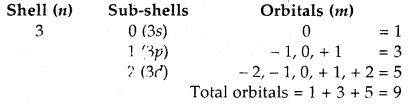### Structure of Atom Chemistry Important Extra Questions Long Answer Type

Question 1.
Describe the shape of s – and p – orbitals What do you mean by node or nodal surface?
Shapes of Orbitals:
s-orbitals: These are spherically symmetrical and non-directional. Shapes of 1s and 2s orbitals are shown in Fig. The effective volume of 2s orbital is larger than Is orbital. Another important feature of 2s orbital is that there is a spherical shell within 2s (region without dots) where the probability of finding the electron is zero. This is called a node or a nodal surface. There are (n – 1) nodes in an s-orbital (where n is the energy level).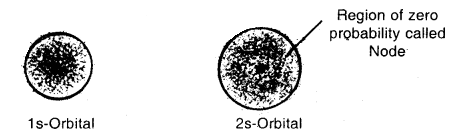p-orbitals: There are three p-orbitals designated as px’, py’ or pz’ which are oriented along the three mutually perpendicular axis x, y, and 2. Each, orbital consists of two lobes symmetrical about the particular axis and has a dumbbell shape. The two lobes are separated by a nodal plane.Shapes of three 2p orbitals

The two lobes of each orbital are separated by a plane having x zero electron density. This plane is known as a nodal plane.

Question 2.
How does the Schrodinger wave equation help to understand the probability of finding the electron near the nucleus? What do you mean by an orbital?
Probability Picture of Electrons:
Schrodinger incorporated the requirements of the uncertainty principle and de Broglie’s concept of matter waves and proposed a mathematical equation to describe the behavior of an electron in an atom. The equation was known as the Schrodinger wave equation.

The Schrodinger wave equation is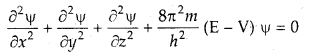where x, y, and z are three space coordinates,
m is the mass of the electron,
h is Planck’s, constant

E is the total energy and V is the potential energy of the electron, φ (Greek letter psi) is the amplitude of the wave, called wave function, $$\frac{\partial^{2} \psi}{\partial x^{2}}$$ refers to the second derivative of φ with respect to x only and so on.

The solution of this equation gave the mathematical expression which gives information about the various energy states and other measurable properties such as the radiation frequencies emitted or absorbed for the hydrogen atom. The solutions of the Schrodinger wave equation are called wave functions and are denoted by the symbol φ.

The physical significance of wave function: In the physical sense φ gives the amplitude of the wave associated with the electron. We know that in the case of light waves, the square of the amplitude, of the wave at a point is proportional to the intensity of light. Extending the same concept of electron wave motion, the square of the wave function, φ2 may be taken as the intensity of electrons at any point.

In other words, φ2 determines the probability density. Thus, φ2 has been called the probability density and φ the probability amplitude. Thus, the solutions of the Schrodinger wave equation replace the discrete energy levels or orbits proposed by Bohr and led to the concept of the most probable regions in space in terms of φ22.

A large value of φ2 means a high probability of finding the electron at that place and a small value of φ2 means low probability. If φ2 is almost zero at a particular point, it means that the probability of finding the electron at that point is negligible. Therefore, the wave mechanics approach gives meaningful wave functions which describe the position and energy levels of electrons in an atom.

Concept of Orbital: An orbital is a region in space around the nucleus where the probability of finding the electrons is maximum.

### Structure of Atom Chemistry Important Extra Questions Numerical Problems

Question 1.
How many nucleons are present in an atom Nobelium, No? How many electrons are present in the atom? How many nucleons may be considered neutrons?
Nucleons = 254, electrons = 102 and neutrons 254 – 102 = 152.

Question 2.
Complete the following table:Question 3.
Complete the following table:Question 4.
Find the number of protons, electrons an-d neutrons in
(a) 1327A3+
p = 13, ē = 10, n = 14

(b) 815O2-
p = 8, ē = 10, n = 7.

Question 5.
Name the element whose atomic nucleus does not contain any neutrons.
The nucleus of the hydrogen atom does not contain any neutron.

Question 6.
Calculate the total no. of electrons present in one mole of methane.
1 Molecule of methane (CH4) contains electrons = 6 + 4 = 10
1 Mole, i.e., 6.022 × 1023 molecules will contain electrons = 6.022 × 1024

Question 7.
Find (a) the total number and
(b) the total mass of neutrons in 7 mg of 14C
(Assume that the mass of a neutron = 1.675 × 10-27 kg).
1 mol of 14C = 6,022 × 1023 atoms
i. e. 14 g of 14C = 6.022 × 1023 atoms

∴ 14 g of it = 6.022 × 1023 × 0.008 neutrons [∵ one atom ofuC contains = 14 – 6 = 8 neutrons]
and now 0.007 g of it will have
= 6.022 × 1023 × 8 × 0.007/13
= 2.409 × 1021 neutrons .

(b) Mass of 1 neutrons = 1.675 × 10-27 kg
∴ Mass of 2.409 × 1021 neutrons = 4.035 × 10-6 kg.

Question 8.
A particular radio station broadcasts at a frequency of 1120 kHz (kilohertz). Another radio station broadcasts at a frequency of 98.7 MHz (megahertz). What are the wavelengths of the radiations from each station?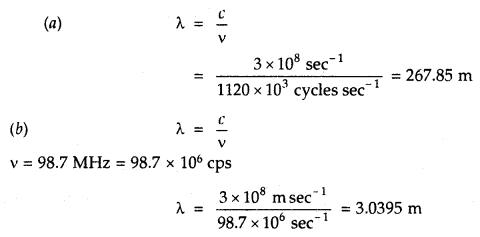Question 9.
Calculate the wave number of radiations having a frequency of 4 × 1014 Hz.
v = 4 × 1014 Hz = 4 × 1014 sec-1
Wave lemgth v̅ = $$\frac{1}{\lambda}=\frac{v}{c}$$
= $$\frac{4 \times 10^{14} \sec ^{-1}}{3 \times 10^{8} \mathrm{msec}^{-1}}$$
= 1.33 × 106 m-1
= 1.33 × 104 cm-1.

Question 10.
A photon of wave length 4 × 10-7 m strikes on metal surface, the work function of the metal being 2.13 eV.
Calculate
(i) the energy of the photon (eV)
Energy of the photon = E = hv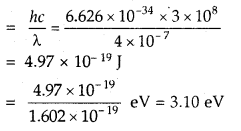(ii) the kinetic energy of the emission and
Kinetic energy of emission = $$\frac{1}{2}$$ mv2 = hv – hv0
= 3.10 – 2.13 = 0.97 eV

(iii) the velocity of the photoelectron [leV = 1.602 × 10-19 J].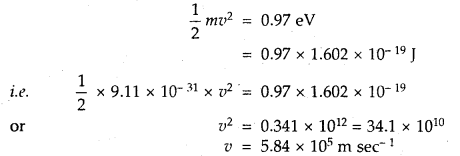Question 11.
Electromagnetic radiation of wavelength 242 nm is just sufficient to ionize the sodium atom. Calculate the Ionisation energy of solution in kJ mol-1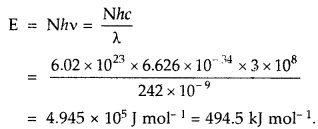Question 12.
The wavelength of the first line in the Balmer Series is 656 nm. Calculate the wavelength of the second line and limiting line in the Balmer series.
According to Rydberg’s formulaFor the Balmer series n1 = 2 and for the first line n2 = 3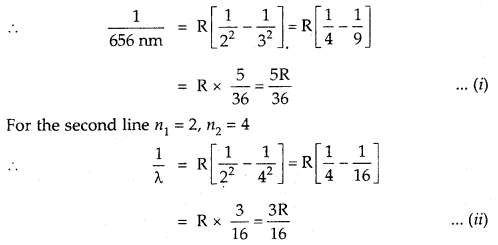Divide (i) by (ii)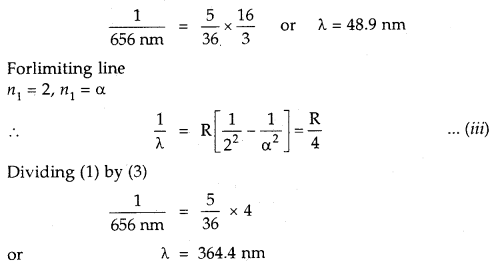Question 13.
Calculate the wavelength of the spherical line in the Lyman series corresponding to n2 = 3.
For the Lyman series n1 = 1
∴ v̅ = R$$\left[\frac{1}{1^{2}}-\frac{1}{3^{2}}\right]$$
= 109577 × $$\frac{8}{9}$$ = 97490.7 m-1
λ = $$\frac{1}{v}=\frac{1}{97490.7}$$ cm-1
= 102.6 × 10-7cm
= 102.6 nm

Question 14.
Calculate the velocity of electrons in the first Bohr orbit of the hydrogen atom. Given that Bohr’s radius = 0,529 A. Planck’s constant h = 6.626 × 10-34 Js mass of electron = 9.11 × 10-31 kg and 1 J = 1 kg m2 s-1.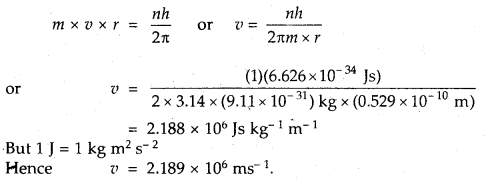Question 15.
The electron energy in a hydrogen atom is given by EH = (- 2.18 × 10-18)/n2 J. Calculate the energy required to, remove the electron completely from n = 2 orbit. What is the longest wavelength of light in cm that can be used to cause this transition?Question 16.
Give the values of the quantum numbers for the electron with the highest energy in the sodium atom.
Electronic configuration of riNa = 1s2, 2s22p6 3s1
The electron with the highest energy is 3s1 for which n = 3 and l = 0

Question 17.
Find the number of unpaired electrons in Fe2+ and Fe3+. At.No. of Fe = 26.
Electronic configuration of 26Fe = 1s2, 2s22p6, 3s2 3p6 3d6 4s2
Fe2+ = 1s2, 2s2 2p6, 3s2 3p6 3d6 It has 4 unpaired electrons
Fe3+ = 1s2, 1s2 2p6, 3s2 3p6 3d5 It has 5 unpaired electrons.

Question 18.
What atoms are indicated by the following electronic cofigurations?
(i) 1s2, 2s22p1,
Total no. of electrons = 2 + 2 + 1 = 5
∴ At. no. of element is 5.
Hence the element is Boron (B).

(ii) [Ar] 4s2 3d1
Total no. of electrons in [Ar] 4s2 3d1 = 18 + 2 + 1 = 21
∴ At. no. of element is 21.
Hence the element is Scandium (Sc).

Question 19.
Give the electronic configuration of N-3, K+, P-3, O2-.

• N = 7 = 1s2,2s2 2p3
• N3- = 10e = 1s2, 2s2 2p6
• K = 19 = 1s2, 2s2 2p6, 3s2 3p6 4s1
• K+ = 18e = 1s2, 2s2 2p6, 3s2 3p6
• P = 15 = 1s2, 2s2 2p6, 3s2 3p3
• p3- = 18e = 1s2, 2s2 2p6, 3s2 3p6
• O = 8 = 1s2, 2s2 2p4
• O2- = 10e = 1s2, 2s2 2p6

Question 20.
Which out of Cu2+, Fe2+, Cr3+ has the highest paramagnetism and why?
Paramagnetism is a property of unpaired electrons. More the no. of unpaired electrons, more the paramagnetism.
Cu2+ = 1s2, 2s2 2p6, 3s2 3p6 3d9
∴ It has only one unpaired electron.
Fe2+ = 1s2, 2s2 2p6, 3s2 3p6 3d6

∴ It has 4 unpaired electrons.
Cr3+ = 1s2, 2s2 2p6, 3s2 3p6 3d3

∴ It has 3 unpaired electrons.
Hence Fe2+ with 4 unpaired electrons is having the highest paramagnetism.

Question 24.
Find the velocity (in ms-1) of the electron in the first Bohr orbit of radius a0. Also, find the de Broglie wavelength in m. Find the orbital angular momentum of 2p orbital of hydrogen in units of $$\frac{h}{2 \pi}$$
Vn = 2.188 × 106 × $$\frac{Z}{n}$$ ms-1
∴ de Broglie wavelength λ = $$\frac{h}{mv}$$
= $$\frac{6.626 \times 10^{-34}}{9.1 \times 10^{-31} \times 2.188 \times 10^{6}}$$ m
∴ Orbital angular momentum = $$\sqrt{l(l+1)} \frac{h}{2 \pi}$$
∴ Orbital angular momentum = $$\sqrt{l(l+1)} \frac{h}{2 \pi}$$ = –$$\sqrt{2} \frac{h}{2 \pi}$$ .